## Friday, April 27, 2012

### Calculus Revisited #7: Finding Extrema

We have arrived at the 7th in a 21 blog entry in our Calculus Revisited series.

Today - we will use the derivative to find local extrema. Extrema include the maximum and minimum values of a function f(x). Finding extrema determines where the function f(x) "turns".

First Derivative Test: A process for finding extreme of the function f(x):

1. Find the roots of f'(x). These roots are called critical points. Let c be a critical point.
2. Compute f'(x) where x is near c but not at x = c.

(A) If f'(x) < 0 when x < c and f'(x) > 0 when x > c, then f(x) has a minimum at x = c.

(B) If f'(x) > 0 when x < c and f'(x) < 0 when x > c, then f(x) has a maximum at x = c.

(C) If (A) or (B) do not apply, then f(x) has an inflection point at x = c.

Second Derivative Test: Another process, this one involving the second derivative:

1. Find the roots of f'(x). These roots are called critical points. Let c be a critical point.
2. Calculate f''(c) (second derivative).

(A) If f''(c) >0, then f(x) has a strict local maximum.

(B) If f''(c)<0, then f(x) has a strict local minimum.

(C) If (A) or (B) do not apply, the second derivative test is inconclusive.

Some observations:

1. If f'(x) > 0, f(x) is said to be increasing at x.
2. If f'(x) < 0, f(x) is said to be decreasing at x.
3. If f''(x) > 0, f(x) is said to be concave up at x. (think of a bowl holding water)
4. If f''(x) < 0, f(x) is said to be concave down at x. (think of a bowl turned upside down)

Problems
1. Find extrema of the function y(x) = -x^3 + 2x + 1

Find the critical points
y'(x) = 0
d/dx (-x^3 + 2x + 1) = 0
-3x^2 + 2 = 0
-3x^2 = -2
x^2 = 2/3
x = ±√(2/3)

The critical points are x = √(2/3) and x = -√(2/3).

By the second derivative test:
y''(x) = -6x

y''(√(2/3)) = -6 * √(2/3) < 0. f(x) has a maximum at x = √(2/3).

y''(-√(2/3)) = -6 * -√(2/3) = 6 * √(2/3) > 0. f(x) has a minimum at x = √(2/3).

√(2/3) ≈ 0.81650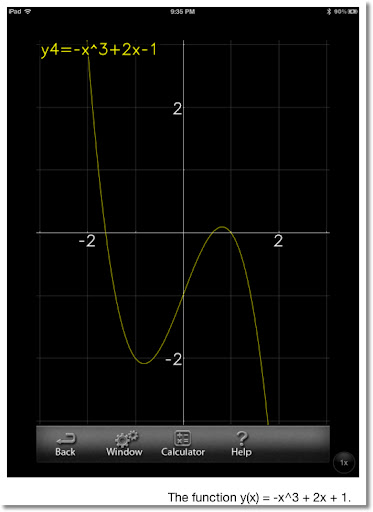2. An open box will be made out of a 12" x 10" sheet of cardboard. (see drawing below, not drawn to scale). A square of length x inches will be cut from each corner of the sheet. What should be the dimensions to construct a box with maximum volume?

x is going to be restricted. First of all x > 0. Second, x < 5. (because 10 - 2 * 5 = 0).

The volume of the box is:
V = (12 - (x + x)) * (10 - (x + x)) * x
= (12 - x^2) * (10 - 2x) * x
= 4x^3 - 44x^2 + 120x

To find the maximum volume, find the critical points. Use the derivative of V in terms of x.

dV/dx = 0
12x^2 - 88x + 120 = 0
x = (88 ± √(88^2 - 4*12*120))/(2*12)
where:
x ≈ 1.8107 and x ≈ 5.5226.

The latter measurement does not make sense since 10 - 2*5.5226 < 0, and negative dimensions do not make sense.

Test point x ≈ 1.8107.

The second derivative:
d^2V/dx^2 = 24x- 88

Then 24(1.8107) - 88 < 0. The test point is a local maximum.

The dimensions of this box are approximately 8.3786'' x 6.3786'' x 1.8107'' with a volume of about 96.7706 in^3.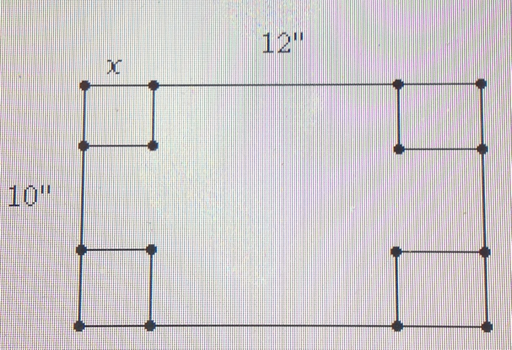3. Minimize the distance between the point (3,1) and the line y(x) = 2x + 5. (see diagram below).

The distance formula between two points (x1, y1) and (x2, y2).

D = (x2 - x1)^2 + (y2 - y1)^2

Find the distance between (3,1) and (x, 2x+5).

D = (x - 3)^2 + (2x + 5 - 1)^2
= (x - 3)^2 + (2x + 2)^2
= 5x^2 + 2x + 13

Taking the derivative D'(x)

D'(x) = 0
10x + 2 = 0
10x = -2
x = -1/5

Since D''(x) = 10 > 0, the point x is a strict local minimum.

The minimum distance is 5 * (-1/5)^2 + 2 * (-1/5) + 13 = 68/5 = 13.6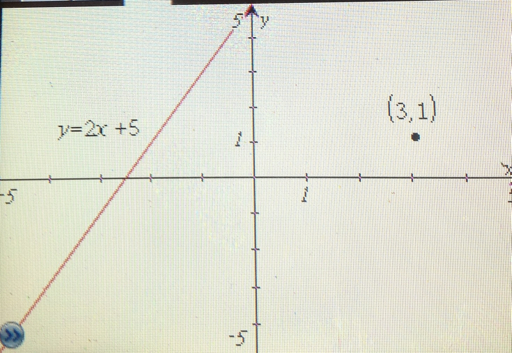Next time we deal with problems involving related rates. Until next time, Eddie.

This blog is property of Edward Shore. © 2012

### Calculus Revisited #6: The Chain Rule

Today we cover a very useful rule in Calculus: The Chain Rule. The rule deals with so many applications in higher mathematics. This is entry #6 in a series of 21.

The Chain Rule
d/dx f(g(x)) = f'(g(x)) * g'(x) = df/dg * dg/dx

If you learn nothing else in calculus - learn this rule! We will do some practice problems with chain rule today.

Problems
1. d/dx (3x^2 + 1)^2

Using the rule above, let f = g^2 and g = 3x^2 + 1.

Then d/dx f(g) = df/dg * dg/dx,
f = g^2
df/dg = 2g
g = 3x^2 + 1
dg/dx = 6x

Putting it all together:
d/dx (3x^2 + 1)^2 = 2g * 6x = 2(3x^2 + 1) * 6x = 36x^3 + 12x

2. d/dx cos(2x^3 - 1)

Let f = cos g and g = 2x^3 -1
Then:
df/dg = -sin g
dg/dx = 6x^2

Put it together:
d/dx cos(2x^3 - 1) = -sin g * 6x^2 = -sin(2x^3 -1) * 6x^2 = -6x^2 * sin(2x^3 - 1)

3. d/dx √(e^x + 1)

Note √x = x^(1/2)

Let f = √g and g = e^x + 1
Then:
df/dg = 1/2 * 1/√g
dg/dx = e^x

So:
d/dx √(e^x + 1) = 1/2 * 1/√g * e^x = e^x / (2 * √(e^x + 1))

Now that you get the though process, let's try another one.

4. d/dx cos^3 x = d/dx (cos x)^3

d/dx (cos x)^3 = 3 * (cos x)^2 * d/dx cos x = 3 * (cos x)^2 * -sin x = - 3 cos^2 x sin x

5. Evaluate the slope of the function y(x) = ln sin x at x = π/4

d/dx ln sin x
= 1 / sin x * d/dx (sin x)
= 1 / sin x * cos x
= cot x

To find the slope at x = π/4, find cot π/4 = 1.

6. The Chain Rule can be applied more than once. This problem illustrates it. Find d/dx ln (cos √x)

d/dx ln (cos √x)
= 1/(cos √x) * d/dx (cos √x)
= 1/(cos √x) * (-sin √x) * d/dx (√x)
= (-sin √x)/(cos √x) * 1/(2√x)
= (-tan √x)/(2√x)

Next time, we will find the extremes of functions.

This blog is property of Edward Shore. © 2012

### Calculus Revisited #5: Derivatives

Welcome to entry #5 of 21 in our Calculus Revisited series. Today, we tackle derivatives!

First the formal definition.

Derivative:

df/dx = f'(x) =

lim ( f(x + δx) - f(x) ) / δx
δx → 0

Note that both symbols for derivatives, the quotient-like symbol and the use of the prime symbol are used interchangeably.

Use the derivative to:
1. Find the instantaneous rate of change at point x0 using the function f(x).
2. Find the slope at given point.
3. When given an equation dealing with the position of an object, f(t), you can find the velocity of an object by calculating f'(t).

But we are going to jump into doing the derivatives. Here is a basic of derivatives:

d/dx ( f(x) + g(x) ) = f'(x) + g'(x)
d/dx ( f(x) * g(x) ) = f'(x) * g(x) + f(x) * g'(x)
d/dx ( f(x) / g(x) ) = (g(x) * f'(x) - g'(x) * f(x))/(g(x)^2)
d/dx a = 0 (a is a constant)
d/dx x = 1
d/dx x^n = n * x^(n-1)
d/dx sin x = cos x
d/dx cos x = -sin x
d/dx tan x = sec^2 x
d/dx e^x = e^x
d/dx a^x = a^x ln a (a is a constant)
d/dx ln x = 1/x
d/dx asin x = 1/√ (1 - x^2)
d/dx acos x = -1/√ (1 - x^2)
d/dx atan x = 1/(x^2 + 1)

TIP: For d/dx ( f(x) / g(x) ), let N = f(x) (numerator) and D = g(x) (denominator). Then d/dx N / D = ( D * N' - D' * N)/(D^2)

Higher Order Derivative: Repeat the derivative operation on f(x).

f''(x) = d^2/dx^2 is the second derivative: take the derivative of f(x), twice.
f'''(x) = d^3/dx^3 is the third derivative: take the derivative of f(x), thrice (three times).
f^(n)(x) = d^n/dx^n is the nth derivative: take the derivative of f(x) n times.

Problems
1. Find the derivative of f(x) = x^2 + 2x + 3.

We can use the addition property to help us.

d/dx (x^2 + 2x + 3)
= d/dx (x^2) + d/dx (2x) + d/dx (3)
= 2x + 2 + 0
= 2x + 2

Remember, the derivative of a constant is 0.

2. Find the slope of f(x) = -x^5 + 2x - 1 at x = 1

First find the derivative
d/dx (-x^5 + 2x - 1)
= d/dx (-x^5) + d/dx(2x) - d/dx(1)
= -5x^4 + 2 - 0
= -5x^4 + 2
The slope is determined by calculating f'(1).
f'(1) = -5(1)^4 + 2 = -3

The slope of f(x) at x = 1 is -3.

3. Find the derivative of f(x) = x^2 * ln x.

Use the product rule: x^2 multiplied by ln x.

d/dx (x^2 * ln x)
= d/dx (x^2) * ln x + x^2 * d/dx (ln x)
= 2x * ln x + x^2 * 1/x
= 2x * ln x + x

4. Find the derivative of f(x) = (x^2 -1)/(x + 2)

Use the division rule: numerator is x^2 -1 and denominator is x + 2.

d/dx ((x^2 - 1)/(x + 2))
= ((x + 2) * d/dx(x^2 - 1) - (x^2 - 1) * d/dx(x + 2))/(x + 2)^2
= ((x + 2) * 2x - (x^2 - 1) * 1)/(x + 2)^2
= (2x^2 + 4x - x^2 + 1)/(x + 2)^2
= (x^2 + 4x + 1)/(x + 2)^2

5. Higher order derivatives: Find the first, second, and third derivative of f(x) = 3x^6 + 7x and g(x) = sin x respectively.

f(x) = 3x^6 + 7x
First Derivative
f'(x) = 18x^5 + 7
Second Derivative
f''(x) = 90x^4
Third Derivative
f'''(x) = 360x^3

g(x) = sin x
g'(x) = cos x
g''(x) = -sin x
g'''(x) = -cos x

6. An interesting property: d/dx( asin x + acos x)

d/dx(asin x + acos x)
= d/dx asin x + d/dx acos x
= 1/√(1-x^2) + (-1)/√(1-x^2)
= 0

This implies that the function asin x + acos x for all x. Bonus question: what is that constant?

Thanks for joining us. Next time we will work with an important rule in calculus: The Chain Rule.

This blog is property of Edward Shore. © 2012

By the way, the answer to the bonus question: asin x + acos x = π/2

## Thursday, April 26, 2012

### Calculus Revisited #4: Limits

Welcome to Calculus Revisited! This is blog entry #4 of a 21 blog entry series. Today, we will cover the basics of limits.

Limit: The limit of a function f(x) is a value of which a function approaches as x creeps closer and closer to it's target x=a. This is not the same as f(a).

In notation:

lim f(x) = L
x→a

The limit may or may not exist.

Left Side Limit: The limit of a function f(x) as x approaches a from the left side. Hence, x < a.

In notation:

lim f(x) = L
x→ a-

Right Side Limit: The limit of a function f(x) as x approaches a from the right side. Hence, x > a.

In notation:

lim f(x) = L
x→ a+

If the left side limit is equal to the right side limit, then the general limit exists at L.

Continuous Function: A function f(x) is continuous if a limit exists for each x in the domain (or specified interval), and that limit is the same as the function's value. It has been often said that you can graph continuous functions without lifting a pencil.

Common continuous functions include:
f(x) = p(x) (polynomials a_n * x^n + ... + a_0)
f(x) = e^x
f(x) = ln x (for x>0 only)
f(x) = sin x
f(x) = cos x

Properties of Limits

lim (f(x) + g(x)) = lim f(x) + lim g(x)

lim c *f(x) = c * lim f(x), c is a constant

Common Ways to Attack Limits

1. If f(x) is continuous, then

lim f(x) = L
x →a

for all x.

2. "Calculator Method": plug in various x_i as x approaches closer and closer to x = a (but not at x = a), observe the results and make a educated conclusion.

3. Graph the function. If graphing calculators are allowed, this is the time to use them.

Problems
1. Find

lim x^2 + 1
x→2

Using the "calculator method":

Left Side Limit: (x approaches 2 with x < 2)
f(2 - .01) = 4.9601
f(2 - .001) = 4.99601
f(2 - .0001) = 4.99960001
f(2 - 10^-9) = 4.999999996
The value is getting close to 5.

Right Side Limit: (x approaches 2 with x > 2)
f(2 + .01) = 5.0401
f(2 + .001) = 5.004001
f(2 + .0001) = 5.00040001
f(2 + 10^-9) = 5.000000004
The value is getting close to 5.

We can reasonably conclude that

lim x^2 + 1 = 5 as x → 2

We could have also observed that x^2 + 1 is continuous everywhere and figured the limit out by plugging in 2 for x.

Now let's go to a case where f(x) is not continuous everywhere.

2. Find

lim 1/(x-2)
x→2

f(2) = 1/0. So plugging in x=2 does not work here.

Left Side Limit: (x approaches 2 with x < 2)
f(2 - .001) = -1,000
f(2 - .0001) = -10,000
f(2 - .00001) = -100,000
f(2 - 10^-9) = -1,000,000,000
Note that f(x) is getting to be a very large negative number, towards -∞

Right Side Limit: (x approaches 2 with x > 2)
f(2 + .001) = 1,000
f(2 + .0001) = 10,000
f(2 + .00001) = 100,000
f(2 + 10^-9) = 1,000,000,000
Note that f(x) is getting to be a very large positive number, towards ∞

But -∞ ≠ ∞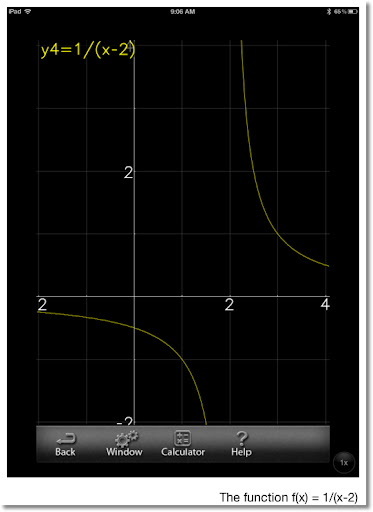3. Find

lim (sin x)/x
x→ 0

Again, f(0) = 0/0, the plugging it won't work.

Left Side Limit:
f(-.001) = .9999998333
f(-.0001) = .9999999983
f(-.00001) = 1 (calculator returns 1)

Right Side Limit:
f(.001) = .9999998333
f(.0001) = .9999999983
f(.00001) = 1 (calculator returns 1)

From the "Calculator Method":

lim (sin x)/x = 1
x → 0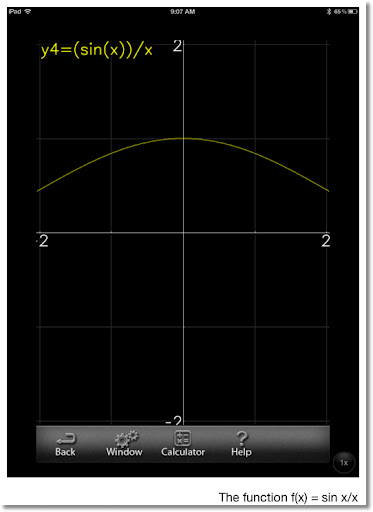The next time we will working with derivatives. See you next time, Eddie.

This blog is property of Edward Shore. © 2012

## Wednesday, April 25, 2012

### Calculus Revisited #3: Logarithmic and Exponential Functions

Welcome to Part 3 of the 21 part series: Calculus Revisited. Today will we talk about exponential and logarithmic functions.

Exponential and Logarithmic Functions

The function y(x) = e^x is a function where the constant e is taken to the power of x. To 25 decimal places, e = 2.7182818284590452353602874. e is known as the Euler's Number or Napier's Constant. (Wikipedia Source)

The function y(x) = ln x is known as the natural logarithmic function (base e), and is the inverse function of the exponential function. In computer mathematical software and Microsoft Excel ln x is referred to as log x.

Note: On calculators, the function log x refers to a common logarithmic function, not natural. log x uses base 10.

Graphs of both e^x and ln x follow.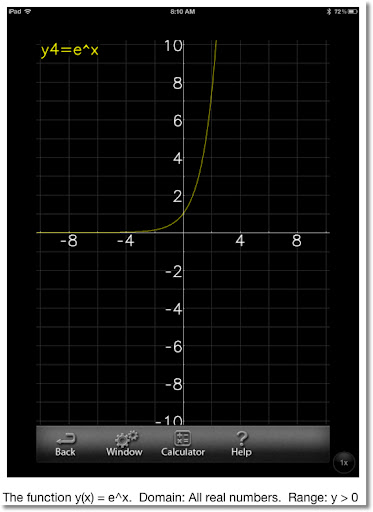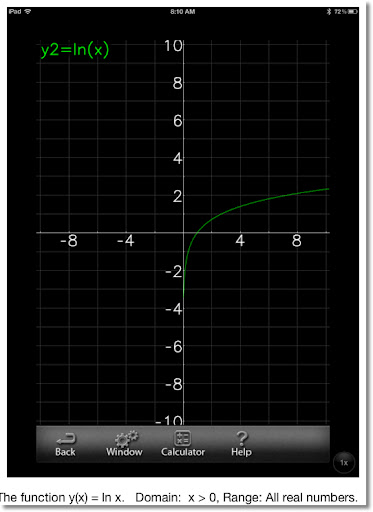Common Properties of the Exponential and Logarithmic Functions

e^x * e^y = e^(x + y)
e^x / e^y = e^(x- y)
(e^x)^y = e^(x * y)

ln(x * y) = ln x + ln y
ln(x / y) = ln x - ln y
ln(x^y) = y * ln x

e^(ln x) = x
ln (e^x) = x

log_n θ = ln θ / ln n (logarithm to base n)

Problems
1. Solve 2^x = 68

2^x = 68
Take the logarithm of both sides
ln (2^x) = ln 68
x ln 2 = ln 68
x = ln 68/ln 2 ≈ 6.08746

2. Solve e^(2x) = 2 * e^(3x)

e^(2x) = 2 * e^(3x)
e^x ≠ 0 for all x, so we can divide.
Divide by e^(2x)
1 = 2 * e^(3x) / e^(2x)
1 = 2 * e^(3x - 2x)
1 = 2 * e^x
1/2 = e*^x
x = ln (1/2) ≈ -.69315

3. Solve e^(2x + 1) = 2^(2x - 3)

e^(2x + 1) = 2^(2x - 3)
ln e^(2x + 1) = ln 2^(2x - 3)
ln e = 1
2x + 1 = (2x - 3) * ln 2
2x + 1 = (2 ln 2)x - (3 ln 2)
(2 - 2 ln 2)x = -3 ln 2 - 1
(2 - 2 ln 2)x = -(3 ln 2 + 1)
x = -(3 ln 2 + 1)/(2 - 2 ln 2) ≈ -5.01778

Next time, we tackle limits. Until next time, take care! Eddie

This blog is property of Edward Shore. © 2012

## Tuesday, April 24, 2012

### Calculus Revisited #2: Working with Trigonometric Functions

Welcome to Part 2 of a series of the 21 Part Series Calculus Revisited, where we cover some of the major topics. Today's and tomorrow's blog entries will be cover the various transcendental functions: today trigonometric functions, tomorrow exponential and logarithmic functions.

Trigonometric Functions

Trigonometric Functions include sine, cosine, and tangent. (abbreviated sin, cos, and tan, respectively) They have corresponding reciprocal functions, cosecant, secant, and cotangent (abbreviated csc, sec, and cot respectively).

The relationship between the trigonometric and their reciprocal functions:

csc x = 1 / sin x
sec x = 1 / cos x
cot x = 1 / tan x

The inverse functions of sine, cosine, and tangent are the arcsine, arccosine, and arctangent functions, respectively. (abbreviated asin or sin^-1, acos or cos^-1, atan or tan^-1 respectively)

sin (asin x) = x
asin (sin x) = x
cos (acos x) = x
acos (cos x) = x
tan (atan x) = x
atan (tan x) = x

The graphs of sin x, cos x, and tan x follow.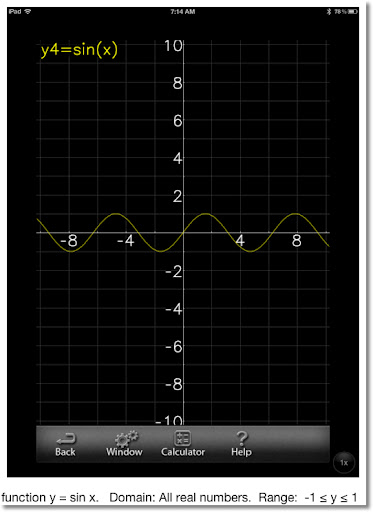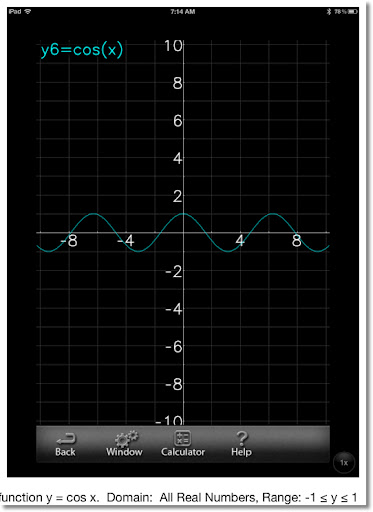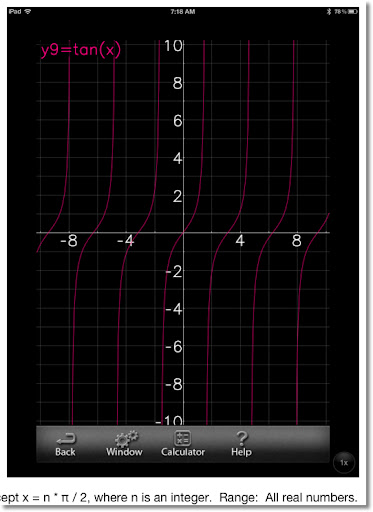Remember: sin^2 x = (sin x)^2. sin^-1 x = asin x. This applies for all trigonometric functions.

More Common Trig Identities

tan x = sin x/cos x
cot x = cos x/tan x

sin^2 x + cos^2 x = (sin x)^2 + (cos x)^2 = 1
tan^2 x + 1 = sec^2 x
1 + cot^2 x = csc^2 x

sin(π - x) = sin x
cos(π - x) = -cos x

sin 2x = 2 sin x cos x
cos 2x = 1 - 2 sin^2 x

sin(a + b) = cos a sin b + sin a cos b
cos(a + b) = cos a cos b - sin a sin b

Problems

1. Let y(x) = a sin (bx), where a and b are any real numbers. What is the significance of a and b?

a is the amplitude of the sine function, where a is the maximum value of y(x) and -a is the minimum value of y(x).

b involved in the period of y(x). The period is π/b.

Note: The variables of a and b have the same significance in the function y(x) = a cos (bx).

2. Is sin x an even or odd function? What about cos x and tan x?

The function f(x) is an even function if for every x, f(-x) = f(x). The function f(x) is an odd function if for every x, f(-x) = -f(x).

Using the graphs above as a reference, sin(-x) = -sin x and cos(-x) = cos x. Note that tan x = sin x/cos x. Then tan(-x) = sin(-x)/cos(-x) = - sin x/cos x = - tan x.

Therefore, sin x is an odd function, cos x is an even function, and tax x is an odd function.

3. Solve cos^2 x - cos x = sin^2 x.

Recall sin^2 x + cos^2 x = 1
Then sin^2 x = 1 - cos^2 x

cos^2 x - cos x = sin^2 x
cos^2 x - cos x = 1 - cos^2 x
2 cos^2 x - cos x - 1 = 0
2 (cos x)^2 - (cos x) - 1 = 0
This is quadratic equation in terms of cos x. This implies that
(cos x - 1) * (2 cos x + 1) = 0
Hence:
cos x - 1 = 0
cos x = 1
x = acos 1 = 0
and
2 cos x + 1 = 0
2 cos x = -1
cos x = -1/2
x = acos (-1/2) = 2π/3

The solutions are x = 0, 2π/3

4. Solve 2 sin x = sin 2x

Recall sin 2x = 2 sin x cos x

2 sin x = sin 2x
2 sin x = 2 sin x cos x
Since sin x can be zero, don't divide by sin x. Instead
2 sin x - 2 sin x cos x = 0
Factor out 2 sin x...
(2 sin x)(1 - cos x) = 0
Then
2 sin x = 0
sin x = 0
x = asin 0 = 0
and
1 - cos x = 0
1 = cos x
x = acos 1 = 0

The solution is x = 0.

Next time we will work exponential and logarithmic functions. Until then, take care! Eddie

This blog is property of Edward Shore. © 2012

## Monday, April 23, 2012

### Calculus Revisited #1: Functions

Welcome to Calculus Revisited

Greetings. Over the next 21 blog entries (Monday - Friday), Eddie's Math and Calculator Blog will revisit and review some of the major topics in one-variable calculus. I may not get to everything, but I am going to hit a lot of the major topics. Let's start off with some pre-calculus and then head into derivatives and integrals. This blog is going to serve as a reference: whether you are a student or a professional. Enjoy, and let's get started!

Sources Used for this Series

Ross, Debra Anne. "Master Math: Calculus" Career Press: Franklin Lakes, NJ 1998

Silverman, Richard A. Ph.D "Calculus with Analytic Geometry" Prentice-Hall Inc., Englewood Cliffs, NJ 1985

With the preliminairies out of the way...

Function Basics

Function: Is an expression (formula or equation) that associates each element of the domain to a corresponding element of the range. A true function is an expression where every element in the domain corresponds to 1 and only 1 element in a range. So no one element in domain can correspond to two (or more) elements in the range.

Domain: The initial set to which a function is applied. The variable that describes the domain is the independent variable.

Range: The set of results. The variable that describes the range is the dependent variable.

So...

domain → Function → range
xy = f(x)y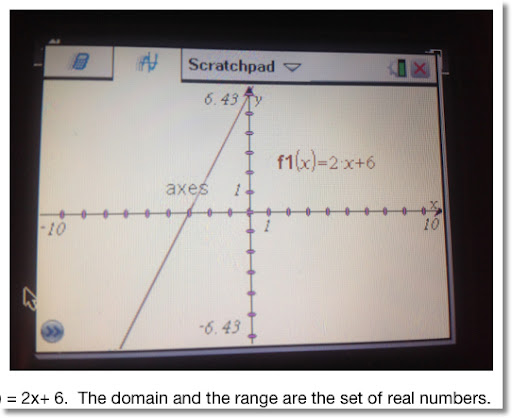Note: For the purpose of this series, I will use x, t, θ (the Greek letter theta) for independent variables and y, f, and g for dependent variables. Obviously any letter or description can be used for variables.

The set of real numbers: that is all numbers including integers, decimals, fractions, and irrational numbers. Real numbers just don't include any complex or imaginary number. So, if √-1 (labeled i or j) is involved, the number is not a real number.

This series will focus exclusively on the set of real numbers.

---------------

1. Find the domain and range of the function y(x) = 2/(x-4).

Note that y(x) is not defined at x=4. Why? y(4) = 2/(4-4) = 2/0. We all know that you can't divide by zero. You can calculate y(x) at various points to verify, however y(x) returns answers that cover the set of real numbers.

Domain: The set of real numbers where x ≠ 4
Range: The set of real numbers

2. Find the domain and range of the function y(x) = √ (16 - x^2).

At first glance, y(x) is defined for all x that make 16 - x^2 nonnegative (positive or zero). To calculate the appropriate domain:

Set:
16 - x^2 ≥ 0
16 ≥ x^2
Take the square root of both sides.
x ≥ -4 and x ≤ 4
The proper domain is
-4 ≤ x ≤ 4

Observe that y(x) hits its maximum point when x = 0
y(0) = √ (16 - 0^2) = √ 16 = 4
Minimum points:
y(4) = √ (16 - 4^2) = 0 and
y(-4) = √ (16 - (-4)^2) = 0

Domain: -4 ≤ x ≤ 4
Range: 0 ≤ y ≤ 4

Composite Functions: Basically, a function of a function. Often noted with a raised circle.

Let f(x) and g(x) be two functions. Then f(g(x)) and g(f(x)) are two composite function. In f(g(x)), the g(x) is treated as the independent variable, and vice versa.

3. Let f(x) = x^2 and g(x) = sin x. Find the composite functions f(g(x)) and g(f(x)).

f(g(x)) = f(sin x) = (sin x)^2 = sin^2 x

Caution: Calculators and most mathematical (if not all) software will not allow the user to enter expressions such as sin^2 x. Use the expression (sin x)^2 instead.

g(f(x)) = g(x^2) = sin (x^2)

Inverse Functions: A function that describes a rule from an element of the range to the element of the domain. If y = f(x), then x = f^-1(y).

4. Let f(x) = 7x + 1. Find the inverse function.

Set y as y = 7x + 1. Solve for x.
y = 7x + 1
y - 1 = 7x
(y - 1)/7 = x

Hence the inverse function is f^-1(y) = (y - 1)/7

Tomorrow: Working with Trigonometric Functions

This blog is property of Edward Shore. © 2012

## Saturday, April 21, 2012

### Review: Casio fx-115 ES PLUS Review

Welcome to blog entry #73. Just an announcement:

Starting Monday on April 23, 2012, I begin my Calculus Revisited Series, where we revisit some of the major topics of calculus. Each blog entry is going to contain a quick summary of the topic, followed by a few example problems. Hopefully this serves as a review for students in calculus in time for finals or perhaps you want to revisit some of the topics. It will be one blog each day, Monday through Thursday except on Friday, where you get three for the price of one. (don't worry, it's free).

Onto today's topic... the Casio fx-115ES Plus Calculator Review!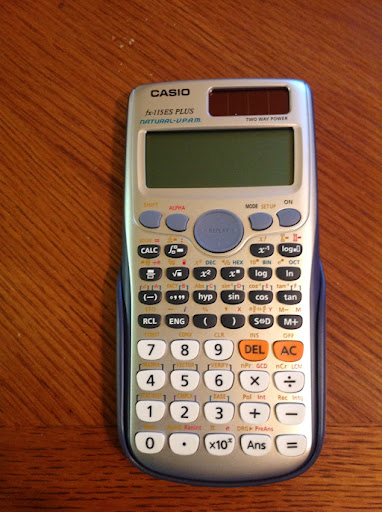The fx-115ES PLUS is the successor to the 2006(?) [when was this calculator first released?] fx-115 ES. Outside of the United States, these calculators are called fx-991ES PLUS and fx-991 ES, respectively.

I purchased the fx-115ES PLUS at a WalMart in Glendora, CA. Apparently, this is the only major store at this time that carries the new fx-115ES PLUS outside of ordering online. It cost me \$17.48, around the same price the classic fx-115 ES cost.

The picture below is a comparison between both models. The new fx-115 is on the left.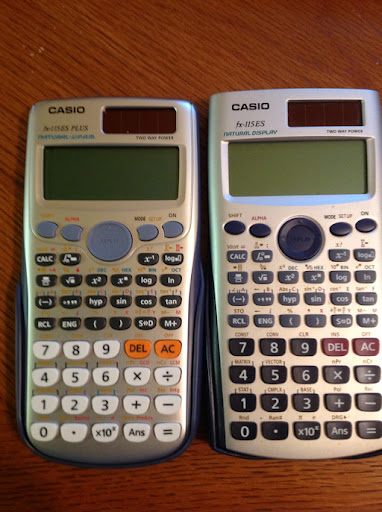Features

The fx-115ES PLUS retains the Natural-V.P.A.M. (Visually Perfect Algebraic Method), which means that you can enter equations and expressions exactly as written. The MathIO method allows the user to accomplish just that. Fractions, radicals, expressions with π, and calculus function templates are all there. Exact answers can include fractions, square roots, and coefficients of π (MathIO).

For those who want a older style, one-line entry method, the LineIO method has been retained. Personally, I prefer the MathIO method.

All of the other features that were present in the classic fx-115 ES are here to:
* Integrals of f(x)
* Numeric Derivatives: d/dx
* Sums of a function: ∑ f(x)
* Base modes Decimal, Octal, Binary, Hexadecimal
* Numeric solver, of equations and roots of expressions. Yes, the variable to solve for is X. (see below)
* CALC button allows for calculating expressions repeated amount of times.
* Statistics including 1-variable, linear regression (a+bx), quadratic regression (a+bx+cx^2), cubic regression (a+bx+cx^2+dx^3), 2 types of exponential (a + b * e^x and a x^b), power (b a^x), logarithmic (a + b ln x), and inverse (a + b/x).
* Equations - 2x2 and 3x3 simultaneous equations, quadratic, and cubic equation
* Matrices: functions include transpose, inverse, and determinant
* Vectors
* Multi Line Statements with the colon (:)
* Complex Number Mode - unfortunately it's still limited to arithmetic, x^2, 1/x.

Hint: To clear calculator history, while in COMP mode, press the [ ON ] key.

Hint: To force approximate answers, press [SHIFT] [ = ]. This works on both versions of the fx-115 ES.

What's New?
* The number of available memories have increased from 7 to 9. (A, B, C, D, X, Y, M, and now E, F). Previously E and F were available only for the Hexadecimal mode.
* You now have the ability to calculate using repeated numbers. For example, you can type the decimal form of 1/3 using 0.3 with the bar above the three. I believe that this is first line of calculators that has this ability.
* New number functions are: GCD, LCM, Integer Part, Fractional Part, Random Integers, Integer Division (÷R) that gives quotient and remainder, and Prime Factorization (up to three digit factors). To factor a number, enter it, press [ = ], then [SHIFT], [ º ' '' ].
* Products of function f(x): ∏ f(x).
* In Table Mode you can include two functions f(x) and g(x). This is turned on in SET UP.
* The [ALPHA] key acts as both as an variable key and a second shift key.
* The rref and ref functions are added to the Matrix Mode (but not eigenvalues).
* The fx-115ES PLUS has a curve design, and boasts a faster processor.

New Modes
* Inequality Solver of quadratic and cubic equations
* Verification mode, used for compare expressions (i.e. Does π/4 < π/2? Does 1 = 9/9 = e^0?)
* Distribution mode: Normal Distribution (CDF, PDF, and Inverse (Yes!)), Binomial Distribution (CDF, PDF), and Poisson Distribution (CDF, PDF).

Final Thoughts
If the fx-115 ES was solid, then the fx-115ES PLUS is taking a great calculator and making it better. At about \$18, you get a huge bang for the buck.

Rating: ***** (5 out of 5)

------------------------------------------------------------------------
UPDATE-CORRECTION

Many thanks to jcroot for pointing this out to me:

The Solve feature can solve for more than just X. You can specify the variable as so:

equation, variable to solve for

If there is no second argument, then the variable to solve for defaults to X.

Example:
X - 2Y = 1, X solves for X
X - 2Y = 1, Y solves for Y
-------------------------------------------------------------------------

This blog is property of Edward Shore. © 2012

## Wednesday, April 18, 2012

### Calculus Revisited # 20: Taylor Series and Maclaurin Series

Welcome to Part 20 of 21: Taylor and Maclaurin Series.

The Taylor and Maclaurin series are representation of the function f(x) by using an infinite series. If we use a finite number of terms, the series can (I stress can), but a good approximation f(x).

A Taylor series of f(x) is centered on a focus point x = a. Generally, approximations are best when x is around a, and gets worse the further x gets from a.

Taylor Series:

About the point x = a:

f(x) = f(a) + f'(a) * (x - 1) + f''(a) * (x - a)^2 / 2! + f'''(a) * (x - a)^3 / 3! + ....

If the series is cut off at n terms, the final term of the Taylor series is:

f^(n+1)(t) / (n+1)! * (x - a)^(n+1). This is known as the error term.

Maclaurin Series:

The Taylor series with a = 0.

f(x) = f(0) + f'(0) * x + f''(0) * x^2/2! + f'''(0) *x^3/3! + ...

With error term f^(n+1)(0) / (n+1)! * x^(n+1)

Some famous series:

e^x = 1 + x + x^2/2! + x^3/3! + x^4/4! + .... + x^n/n! + ....

sin x = x - x^3/3! + x^5/5! - x^7/7! + .... + ( (-1)^n * x^(2n+1) ) / (2n+1)! + ....

cos x = 1 - x^2/2! + x^4/4! - x^6/6! + ... + ( (-1)^n * x^(2n) ) / (2n)! + ....

Problems

1. Find a Macluarin series for

f(x) = ln(x + 1) to four terms

f(x) = ln(x + 1)
f(0) = ln(0 + 1) = 0

f'(x) = 1/(x+1)
f'(0) = 1/1 = 1

f''(x) = -1/(x+1)^2
f''(0) = -1/(1^2) = -1

f'''(x) = 2/(x+1)^3
f'''(0) = 2/(1^3) = 2

Then:

ln(x + 1) = 0 + 1 * x + (-1) * x^2/2! + 2 * x^3/3! + ....
= x - x^2/2! + 2x^3/3! + ....

2. Find the Macluarin series for

f(x) = e^(-x^2) to three nonzero terms. Approximate its integral.

f(x) = e^(-x^2)
f(0) = 1

f'(x) = e^(-x^2) * (-2x)
f'(0) = 0

f''(x) = e^(-x^2) * (4x^2 - 2)
f''(0) = -2

f'''(x) = e^(-x^2) * (-8x^3 + 12x)
f'''(0) = 0

f''''(x) = e^(-x^2) * (16x^4 - 48x^2 + 12)
f''''(0) = 12

Then:

e^(-x^2) = 1 - 2 * x^2/2! + 12 * x^4/4! + ....
= 1 - x^2 + x^4/2 + ...

∫ e^(-x^2) dx = x -x^3/3 + x^5/10 + .... + C

3. Find the Taylor series for cos x at a = π/4 to three nonzero terms.

f(x) = cos x
f(π/4) = √2/2

f'(x) = - sin x
f'(π/4) = -√2/2

f''(x) = -cos x
f''(π/4) = -√2/2

Then:

cos x = √2/2 - √2/2 * (x - π/4) + √2/2 * (x - π/4)^2/2! + ....

That concludes our section on Taylor and Maclaurin series. The next and last entry will be our "catch all" entry.

Thank you as always,

Eddie

This blog is property of Edward Shore. © 2012

## Tuesday, April 17, 2012

### Announcement: Calculus Revisited starts 4/23/2012

Blog Entry #72

Starting on April 23, 2012, I will revisit topics of calculus for 21 posts (my goal is to post daily - at least on Monday - Friday). It can be a used for a review, visiting past (hopefully pleasant) memories, or an aid for studying for the finals.

I will cover some of the major topics in calculus including:
* Trigonometric Identities
* Functions
* Limits
* Derivatives
* Integrals
* Series
* Entry 21 will be a "catch all" blog

See you soon!

Eddie

This blog is property of Edward Shore. © 2012

## Monday, April 16, 2012

### Calculus Revisited #10: Integration Basics

Welcome to Part 10 of our 21 Calculus Revisited series. Today, we enter the world of Integration.

A function f(x) has an antiderivative F(x) if the following is true:

∫ f(x) dx = F(x) and d/dx F(x) = f(x)

Indefinite Integral: Finding a symbolic integral of f(x) to describe all the antiderivatives of f(x).

In a calculus course, if F(x) is the antiderivative of f(x), then

∫ f(x) dx = F(x) + C

where C is a numerical constant. Calculators with CAS (computer algebraic system) capabilities usually do not include the C when figuring out indefinite integrals. It is not technically wrong, the CAS system assumes that C = 0, most of the time.

Definite Integral: An integral that has a limit from a
to b (usually a < b, but not always) which produces a numerical answer. The most famous example of a definite integral is the area under the curve f(x) from a to b.

By the Fundamental Theorem of Calculus,

b
∫ f(x) dx = F(b) - F(a)
a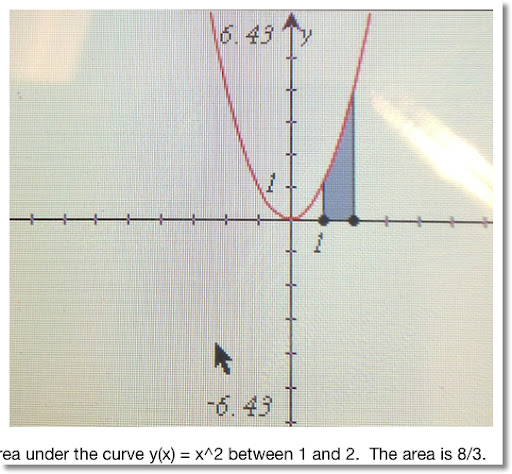Table of Integrals

∫ f(x) + g(x) dx = ∫ f(x) dx + ∫ g(x) dx

∫ a dx = a * x (a is a constant)
∫ x^n dx = (x^(n+1)) / (n+1)
∫ 1/x dx = ln x
∫ e^x dx = e^x
∫ sin x dx = -cos x
∫ cos x dx = sin x
∫ tan x dx = -ln |cos x|
∫ asin x dx = x asin x + √(1 - x^2)
∫ acos x dx = x acos x - √(1 - x^2)
∫ atan x dx = x atan x - ln(x^2 + 1)/2
∫ 1/√(1 - x^2) dx = asin x
∫ 1/(1 + x^2) dx = atan x
∫ 1/√(x^2 - 1) dx = ln(√(x^2 - 1) + x)
∫ a^x dx = a^x/(ln a)

Problems
Indefinite Integrals

1. ∫ 2x^2 + x - 1 dx

∫ 2x^2 + x - 1 dx
= ∫ 2x^2 dx + ∫ x dx - ∫ 1 dx
= 2/3 * x^3 + C + x^2/2 + C - x + C
C just symbolizes a general constant, do not worry if it is 3C or just C in this case
= 2/3 * x^3 + x^2/2 - x + C

In the future, for indefinite integral problems, we will just add the C at the end.

2. ∫ 1/x^2 - 1/x dx
= ∫ x^(-2) dx - ∫ 1/x dx
= -1/x - ln x + C

3. ∫ 2 * 3^x dx

Note: ∫ a * f(x) dx = a * ∫ f(x) dx. Know this - it will come in handy a lot.

∫ 2 * 3^x dx
= 2 * ∫ 3^x dx
= 2 * (3^x)/ln 3 + C

Definite Integrals

Hint: When using a calculator to calculate definite integrals - as a general rule, always have your calculator in Radians mode.

4.

1
∫ 1/(√(1 - x^2) dx
0

Use the Fundamental Theorem of Calculus to assist us with these type of problems.

f(x) = 1/(√(1 - x^2)
F(x) = asin x

1
∫ 1/(√(1 - x^2) dx
0

= asin 1 - asin 0
= π/2 - 0
= π/2

Hint: Do not worry about the "C" with definite integrals, they cancel out!

b
∫ f(x) dx
a

= F(b) + C - (F(a) + C)
= F(b) + C - F(a) - C
= F(b) - F(a)

5.

2
∫ 2 * e^x dx
0

2
= ∫ e^x dx
0

= 2 (e^2 - e^0)
= 2 (e^2 - 1) ≈ 12.77811

( ∫ e^x dx = e^x )

Limits do not have to be constants. Check out the next problem.

6.

t
∫ cos x dx
0

= sin(t) - sin 0
= sin(t)

( ∫ cos x dx = sin x)

Initial Value Problems - Basically, figure out what C is.

7. Find the antiderivative of f(x) = 2x + 5 if the antiderivative passes the point (0, 1).

We are faced with two tasks:
1. Find F(x) in general
2. Find the specific F(x) knowing F(0) = 1. This is where we solve for C.

∫ f(x) dx = F(x) + C

∫ 2x + 5 dx
= x^2 + 5x + C

With F(0) = 1 (going through point (0,1)): (x = 0, y = 1)

1 = (0)^2 + 5 * (0) + C
1 = 0 + C
1 = C

The final answer is F(x) = x^2 + 5x + 1

8. The slope of the function y(x) is described by the function sin x. It is known that y(x) passes through the point (1/2, 1/2). Find y(x).

The slope of the function y(x) is the derivative of y(x), or y'(x).

Then ∫ y'(x) dx = y(x)

And
∫ sin x dx = - cos x + C
Almost there, we have to find what C is. Use the point (1/2, 1/2) to help.
1/2 = - cos(1/2) + C
1/2 + cos(1/2) = C
We can conclude that
y(x) = cos x + (1/2 + cos(1/2)) ≈ cos x + 1.37758

We have just started with integrals. The next four parts will deal with techniques of integration.

See you soon, Eddie

This blog is property of Edward Shore. © 2012

## Saturday, April 14, 2012

### Calculus Revisited #8: Related Rates

In our 8th in our 21 blog entry series, we will deal with some problems involving related rates.

Simply put, if you have a function f(x), then f'(x) describes the rate of change at point x.

In physics, you can describe the position of an object given any point in time by the equation x(t). The velocity is found by the first derivative x'(t), and the acceleration is found by the second derivative x''(t).

Position -(derivative)-> Velocity -(derivative)-> Acceleration

Problems
1. A ball is dropped from a building four stories high (48 feet). Let the position equation be x(t) = -1/2 * g * t^2 + 48 where g is the gravitational constant at 32.17405 ft/s^2. When will the ball hit the ground? What will be the velocity and acceleration when the ball hits the ground?

When will the ball hit the ground? How much time will it take for the ball to hit the ground? Solve x(t) = 0.

-1/2 * 32.17405 * t^2 + 48 = 0
-16.087025 * t^2 = -48
t^2 ≈ 2.98377
t ≈ 1.72736 (negative time is nonsensical)

It takes approximately 1.72736 seconds for the ball to reach the ground.

What is the velocity and acceleration when the ball hits the ground?

Velocity: x'(t) = -g * t
x'(1.72736) ≈ -32.17405 * 1.72736 ≈ -55.57617 ft/sec (velocity of the ball moving downward)

Acceleration: x''(t) = -g
The acceleration is 32.17405 ft/sec^2.

2. A rectangular tub 6 feet wide by 4 feet deep is filled with water with its height increasing at 6/5 in/sec. How fast is the volume of water increasing? If the tub is 3 feet high, when will the tub be full?

1 foot = 12 inches

The tub is 72 inches wide by 48 inches deep by 36 inches high. The rate of the increase in height in water is 1.2 in/sec.

Volume of the water = width of the tub * depth of the tub * height of the water

Note that width and depth do not change, only the height.

Then:
V_water(t) = 72 * 48 * h(t)
= 3456 * h(t)
Take the derivative with respect to t:
V_water'(t) = 3456 * h'(t)
The water is rising at 1.2 in/sec. Hence h'(t) = 1.2
V_water'(t) = 3456 * 1.2 = 4147.2

The volume of water is increasing at a rate of 4,147.2 in^3/sec, or 2.4 ft^3/sec.

When will the tub be filled?

The tub will be filled when the height of the water reaches 3 feet, or 36 inches.

distance = rate * time
height of tub = h'(t) * time
36 = 1.2 * t
t = 30

The tub will be full in 30 seconds.

3. Let q(t) = 1/3 * t^3 - t^2 + t coulombs of charge flow through a conducting wire with time (t) being measured in seconds. What is the current after 4 seconds? If there is a 25-amp fuse in the wire, how long will it last?

In electronics, the current of the rate of charge. Current is symbolized by i(t).

Then i(t) = q'(t).

So i(t) = d/dt (1/3*t^3 - t^2 + t) = t^2 - 2t + 1

And i(4) = 16 - 8 + 1 = 9

The current is 9 amp (coulombs/sec).

If there is a 25-amp fuse, how long will it last?

The fuse burns out when the current is 25 amps. So we are solving for t.

i(t) = 25
t^2 - 2t + 1 = 25
t^2 - 2t - 24 = 0
( t - 6 ) ( t + 4 ) = 0

This implies that t = 6 (negative time is nonsensical).

The fuse lasts for 6 seconds.

This concludes Part 8 of this 21 part series. Next time, we will solve non-linear equations.

In good calculation, Eddie.

This blog is property of Edward Shore. © 2012

## Friday, April 13, 2012

### How Far Scientific Calculators Have Come

Happy Friday the 13th - Blog Entry # 71

How Far Scientific Calculators Have Come

To put it briefly, the Casio fx-115 ES is a Casio 9860g minus the programming and graphing.

The TI-36X Pro is a TI-84 Plus minus the programming and graphing.

Both have definite integrals, numeric derivatives, numeric solvers, polynomial solvers, (some) complex number operations, statistics, base operations, a library of constants and conversions, and Σ (sum of a series).

40 years ago we were looking at getting just trigonometry and logarithms for \$395. (HP 35). (Thank you Hewlett Packard for making the scientific calculator part of our lives. And for bringing back the HP 15C. )

I am excited about the new Casio fx-115ES Plus that is coming soon. I think the model adds product of a series (Π), GCM, LCD, integer part, and fraction part. (partially to catch up to the TI-36X Pro).

Casio has got to consider expanding the programmable fx-3650P, and marketing it in America.

Can a solar graphing calculator not be far behind? Who would do it first?

What about RPN? I have been forever dreaming of a having an RPN solar calculator.

Announcements

Coming at the end of April, I am anticipating April 30, 2012, I am going to post a review series on one-variable calculus. It will last about a few weeks.

Have a good weekend everyone!

This blog is property of Edward Shore. © 2012

## Sunday, April 8, 2012

### Taking Some Mystery Out Of Baseball Statistics

Welcome to Blog Entry #70.

A shout to all who are following my blog. Many sincere thanks!

This will probably be my first anniversary post, even though it is still about a week away. I started this blog on April 16, 2011.

Today's blog entry will cover baseball statistics. Any one who follows baseball, especially in America, will hear tons of statistics for each player, for every single kind of situation possible. I guess this is how a lot of people keep busy watching the sport.

I am going to go over some of the more common statistics. Apparently the only thing complex about statistics is the amount of statistics and abbreviations and not the mathematics itself.

Baseball Stats

BA: Batting Average

BA = H / AB

The higher, the better.

H = the number of hits a player makes. This is each time a player successfully reaches at least one base after hitting a pitch.

AB = number of at-bats, the number of the times a player appears in front of a pitcher. However an at-bat does not include situations when the batter walks (receives four pitches deemed to be "balls"), the batter is hit by a pitch, or hits a sacrifice (intentionally bats out to get a teammate to score a run).

Examples:
Note: All examples use statistics from the 2011 season - provided by MLB and ESPN. All calculations result in three decimal places.

Matt Kemp, Los Angeles Dodgers: 195 hits in 602 at bats
BA = 195 / 602 = .324

Evan Longoria, Tampa Bay Rays: 118 hits in 483 at bats
BA = 118 / 483 = .244

SLG: Slugging Percentage

SLG = TB / AB = (singles + 2 * doubles + 3 * triples + 4 * home runs) / AB

The higher the better.

TB = total bases, a weighted sum of hits

Matt Kemp: 353 total bases in 602 at bats
SLG = 353 / 602 = .586

Evan Longoria: 239 total bases in 483 at bats
SLG = 239 / 483 = .495

ERA: Earned Run Average

ERA = 9 * ER / IP

The lower, the better.

ER = earned runs scored, in this case, by the opponent. This does not include any runs scored by "errors".

IP = innings pitched. Innings are counted by outs. So if a pitcher threw for six innings and got two outs in the seventh inning, the pitcher has pitched for 6 2/3 innings.

Caution: For IP, some statisticians use shortcut notation. For an IP listed as n.1, it means n and 1/3 innings. (n is a number). If the IP is listed as n.2, it means n and 2/3 innings. Example: A listed IP of 233.1, it means 233 1/3.

Clayton Kershaw, Cy Young Award Winner, Los Angeles Dodgers: 59 earned runs allowed in 233 1/3 innings pitched.
ERA = 9 * 59 / (233 1/3) = 2.276

Chris Carpenter of the 2011 World Series Champions St. Louis Cardinals: 91 earned runs in 237 1/3 innings pitched.
ERA = 9 * 91 / (237 1/3) = 3.451

K/9: Strikeouts Per 9 Innings Pitched

K/9 = 9 * K / IP

The higher, the better.

K = strike out. A pitcher gets this when the pitcher gets the batter to swing and miss on a pitch which results in Strike 3 (symbolized by a forward K), or the pitcher throws the ball to a batter's strike zone but the batter does nothing and is thrown out on Strike 3 (symbolized by a backwards K). Any batter who gets Strike 3 is out.

Per ESPN, Clayton Kershaw's K/9 for 2011 was 9.57 while Chris Carpenter's was 7.24. A K/9 above 9 is considered excellent.

RC: Runs Created (Bill James)

RC = (TB * (H + BB)) / (AB + BB)

The higher the better.

BB = base on balls. A BB is earned when a hitter takes a pitched deemed Ball 4 (the pitcher missed).

This is an estimate of runs created and not a count of the number of runs the player scored.

Paul Konerko, Chicago White Sox: 281 total bases, 163 hits, 77 walks in 543 at bats
RC = (281 * (163 + 77)) / (543 + 77) = 108.774 (estimated 109 runs created)

RBI: Runs Batted In

Commonly known as "ribbys", a batter gets an RBI every time the team scores a run that do not result from "errors". A batter can get up to four RBIs every time the batter is at bat.

Example: A batter has teammates at second and third base. The batter hits a double going to second base, allowing both teammates to reach home base. The batter scores 2 RBIs.

WHIP: Walks and Hits Per Innings Pitched

WHIP = (BB + H)/IP

The lower the better, real good of WHIP is below 1.

C.J. Wilson, was with the Texas Rangers in 2011, now with the Los Angeles Angels of Anaheim in 2012: 74 walks (BB), 191 hits allowed, 233 1/3 innings pitched
WHIP = (74 + 191)/(233 1/3) = 1.187

WAR: Wins Above Placement

Thankfully this is not a measurement of a baseball player's ability to use a weapon. WAR is an encompassing statistic of a player's contribution both offensively and defensively. You may have seen WAR graphs on various sports web sites. Generally, if your WAR is above 8, you are MVP material. If your WAR is around 5, you are an All-Star. If your WAR is around 2, you're an average starter. WAR at 0 and... you will most likely soon to be replaced with a minor leaguer.

These are just some of the statistics that baseball uses. Now it's time to go grab a soda and a hot dog - PLAY BALL!

Source:

MLB Clubhouse - Glossary Page

This blog is property of Edward Shore. © 2012

### Review: iMathPac App from Mobile Caltronics

Welcome to blog entry # 69: Happy Easter to Everyone!

Review: iMathPac by Mobile Electronics

Platforms: iPhone, iPod Touch, iPad (can be zoomed)
Cost: Free
Location of Company: Central Florida

iMathPac is an app that features a scientific calculator and a graphing application. The features iMathPac have are:

* Trigonometry
* Angle Modes included are Degrees and Radians
* Factorial for any number, not just integers
* Permutations and Combinations
* Full complex number support (rectangular notation only)
* Graphing of functions, inequalities, and inverse functions.
* One touch conversion between fractions and decimal approximations

Below are some screen shots of this powerful and free calculator.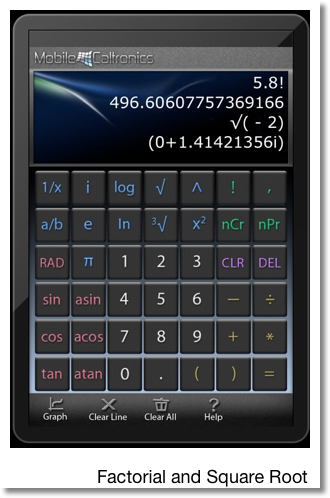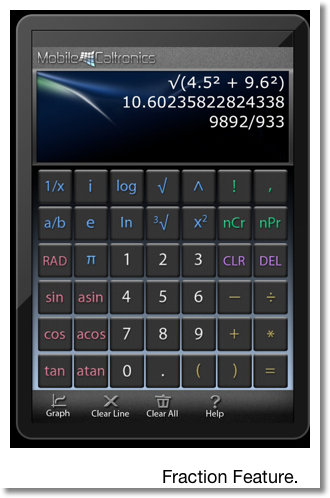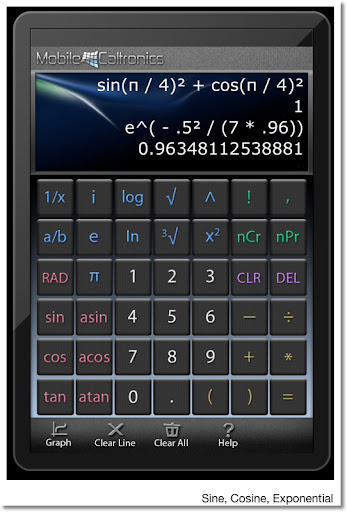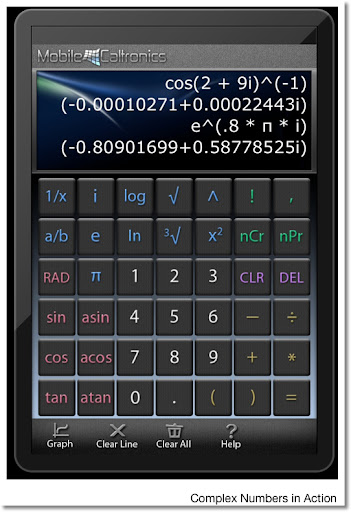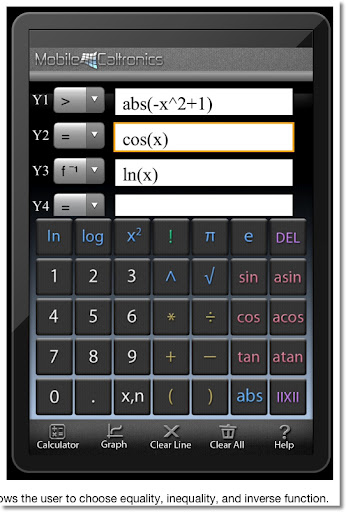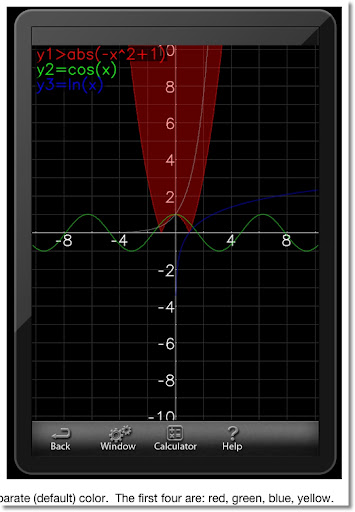Notes:

* The numeric keypad is in the layout of a phone, which is opposite of the key layout on most calculators.
* There is no shift key, which I believe is by the company's design.
* The e key enters the constant e = 2.17828182845905 (to 16 decimal places). To use the exponential function, just press the e key then the ^ key.
* If you press the 1/x, "^(-1)" will be attached to the end of expression.
* Complex number calculations include trigonometric (watch the angle mode), logarithmic, powers and roots, and exponential - which is really nice!
* There is no "EE" key, so you have to enter large exponents as such: nnnn*10^(xxx)
* The minus key, -, acts as both the negation key and subtraction. In calculators with algebraic systems, I prefer this to having separate keys for negation and subtraction.
* The gamma function can be calculated using the factorial: Γ(x) = (x-1)!
* The absolute value (abs) and floor function (||x||) are available in graph mode only. I do believe the graph mode operates in Radians mode only.
* Tap on the graph to find the value of all functions. Pinch to zoom (not perfect).

* Full complex number support - and yes I am a big fan of this
* The factorial function is not restricted to integers - I am also a big fan
* The screen looks sweet, well use of color
* The ability to graph inequalities and inverse functions
* Who can beat free? A lot of features for a free calculator.

What Could be Improved
* No memory, store, and recall. This could be helpful in complex calculations.
* There is no fixed decimal mode settings.
* You can copy expressions, but where to paste them?

Recommendation
iMathPac is a free, powerful calculator, with a lot of powerful features. It is a nice scientific calculator with great graphing capabilities. Definitely worth the download.

Rating: * * * * * (out of 5)

This blog is property of Edward Shore. © 2012

## Tuesday, April 3, 2012

### Pies in the Face and Inelastic Collisions

Welcome to blog entry # 68 of my math and calculator blog!

Before I start, I dedicate this blog entry to pranksters, professional clowns (the ones whose job is it to bring laughs, not the annoying politician kind), Shane Jensen, Chris Brame, A.J. Burnett, the Texas Rangers, and the New York Yankees.

Today I am on the tail end of a nasty cold. The saying "laughter is the best medicine" and the beginning of the 2012 MLB baseball season reminds me of the good old "pie in the face" prank. Yes, I like baseball for a lot of reasons and I have grown to like the pieing prank as part of it.

This blog entry, I attempt to bring math, physics, and cream pies together. For those of you who expect a serious subject, my apologizes, but I am going for the silly side today.

Here is what typically happens when someone throws a pie in... a face. Disclaimer: I do not claim to be an artist by any means.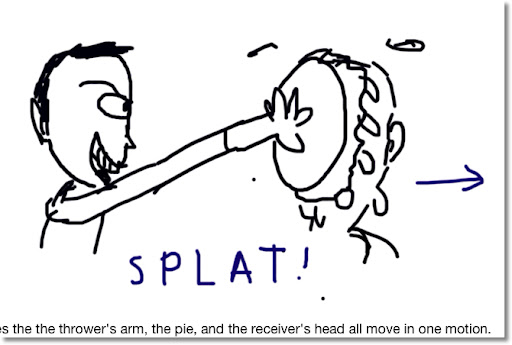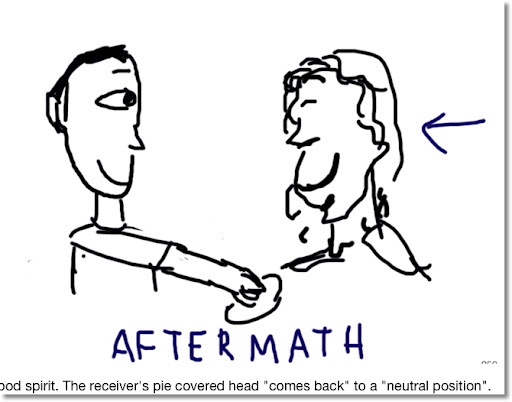So I break the pie in the face into two stages:

1. Before to Splat
2. Splat to Aftermath

Since the pie sticks to the receiver's head, it is safe to say that the pie in the face row is an example of an inelastic collision. Momentum will be preserved but not kinetic energy.

Variables:

m_pie = mass of a cream pie, about 1 to 2 pounds

m_arm = mass of the thrower's arm, which is about 6.5% of a human's weight according to Shirley A Kindrick, PhD of The Ohio State University.

m_head = mass of the receiver's head. It is approximately 8 to 12 pounds according to The Physics Factbook edited by Glenn Elert.

v_t = velocity of the arm throwing the pie

v_f = velocity of the arm, pie, and head after the contact

v_a = velocity of the pie covered head after contact

Assumptions:

1. The entire pie in the face motion is done in one-dimension. That is, back and forth. The receiver is getting the pie head on at no angle.

2. In the Splat to Aftermath stage, the thrower's arm "falls" out of the picture, allowing the reciever's head to come back to neutral.

3. The amount of "pie" lost is negligible when the contact occurs.

4. To keep is problem simple, the thrower does not "smear" the pie over the receiver.

5. Both the thrower and receiver are standing still. The receiver is not trying to duck, avoid, or do anything to avoid being pied. The receiver exerts no effort after being pied.

Momentum Conservation

Before to Splat Stage:

(m_pie + m_arm) * v_t = (m_pie + m_arm + m_head) * v_f

Splat to Aftermath Stage:

(m_pie + m_arm + m_head) * v_f = (m_pie + m_head) * -v_a

v_a is negative since the head is moving "back" after the thrower releases his grip.

Let's try a Numeric Example

I am going to use US Customary units here:

* pounds for mass (lb) [the SI unit is kg]
* feet/second for velocity (ft/s) [the SI unit is m/s]
* foot-pound (lb_f) for a unit of energy [the SI unit is J (Joule)]

Conversion: 1 mi/hr = 22/15 ft/s. (approximately 1.466667)

A thrower, weighing 210 pounds is holding a 1.5 pound pie at 0.6 mph. He launches it on to his friend's face. His friends weights 220 pounds, assuming his head weights 10 pounds. What is the velocity of friends's head at contact and after contact? What is the kinetic energy at the competition of the two stages?

Data:
m_pie = 1.5 lb
m_arm = 6.5% * 210 = 13.65 lbs
v_t = 0.6 mph = 0.88 ft/s

Before to Splat Stage:
v_f:
(1.5 + 13.65) * 0.88 = (1.5 + 13.65 + 10) * v_f
15.15 * 0.88 = 25.15 * v_f
13.33202 = 25.15 v_f
0.53010 = v_f

The arm, pie, and head are moving approximately 0.53010 ft/s (0.77748 mph) at Splat.

Kinetic Energy (KE = 1/2 * mass * velocity^2)
KE before Splat = 1/2 * 15.15 * 0.88^2 ≈ 5.86608 ft_lb
KE at Splat = 1/2 * 25.15 * .53010^2 ≈ 3.53365 ft_lb
(a loss of approximately 39.8%)

Splat to Aftermath Stage:
v_a:
25.15 * .53010 = (1.5 + 10) * -v_a
13.33202 = 11.5 * -v_a
v_a ≈ -1.15931

At the aftermath stage, the velocity of the pied head is 1.15931 ft/s (0.79044 mph) in the other direction, a small whiplash after pushing the friend back with that pie.

Kinetic Energy:
At Aftermath: KE = 1/2 * 11.5 * 1.15931^2 ≈ 7.728 lb_ft
(more than double after release due to loss of mass)

So this is an attempt to explain the physics of the pie in the face.

Thanks for reading as always. To Shane Jansen, Chris Brame and those who like pies in the face, I splat you with a virtual pie.

This blog is property of Edward Shore. © 2012

### TI-Nspire: Templates to Plot Functions, Parametric Equations, and Sequences

TI-Nspire:  Templates to Plot Functions, Parametric Equations, and Sequences Introduction The following are sample templates to plot functi...